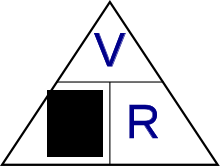top of page
Search

# What is Ohms Law

In this article we are going to discuss what ohms law is. Show you what it is useful for, and of course teach you exactly how to use it.

As an Engineer or Technician you’ll often be working on or designing electronic circuits.

Ohms Law describes the link between Current , Voltage and resistance within these circuits.

If you aren’t familiar with the concepts of Current, Voltage and resistance within electrical circuits there is a useful analogy that makes it all very clear.

Lets take look at the most basic electrical circuit of a battery, some wire and a resistor.The battery creates a potential difference of energy states, or voltage, that causes electrons to flow through the connected wire, (The current) that then travels through the resistor and back to battery.

The analogy that can help us understand this your garden water hose.

Think of the battery or voltage as the pressure of your water supply, The higher the pressure the more force is trying push the water out of your outlet.

The outlet valve is acting as a resistor by reducing the amount of water that can leave the outlet. The flow of water that does leave the outlet and through the hose can be thought of as the current.

All three of these properties are linked, if we increase the resistance or close the outlet valve off, Then the flow is reduced. If we open the valve up, or decrease the resistance. Then the flow increases.

Ohms Law describes this link between Current / resistance and voltage. If we know the value of two of these properties then we can calculate the third using ohms law.

Its worth noting here that in engineering:

• Voltage is denoted as "V" and measured in Volts

• Resistance is denoted by "Ω" or "R" and measured in Ohms

• Current is denoted by "I" and is measured in Amps.

You’ll often see ohms law written as a triangle Like this...From this triangle we can quickly work out the formula for any of the three values by covering the value we are looking for and looking at what we are left with.

If we want to know Volts, we can then cover the volts and here we can see we are left with:

I (Current) x R (Resistance)

If we want to know the Current, we can cover the I and are left with with:

V (Volts) / R (Resistance)

And lastly if we want to know the Resistance we can cover the R and are left with:

V (Volts) / R (Resistance)

Lets say we have a 12V battery and we connect with some wire a 250 ohm resistor across this battery.

Its worth mentioning here that our connecting wire will have a small resistance value also but for this example we will treat the total resistance as 250 ohms.

So we want to know what current is flowing through the circuit without using a meter.

Lets use ohms law to calculate this.

So we look at our triangle and know we want to work out the current. We cover the I and are left with Voltage divided by Resistance.So we take 12volts and divide this by 250 ohms and that gives us 0.048A. which can also be written as 48mA. All this without using a meter:

12 / 250 = 0.048

If you have a play around with the other values in this circuit and see if it makes sense to you

Hopefully you now have a better understanding of ohms law and what it can be used for.